Next: 17.7 Rational Functions Up: 17. Antidifferentiation Techniques Previous: 17.5 Trigonometric Substitution   Index

# 17.6 Substitution in Integrals

Let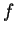be a nice function on an interval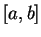. Then if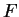is any antiderivative for, we have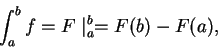by the fundamental theorem of calculus. We saw in (17.32) that under suitable hypotheses on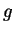,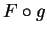is an antiderivative for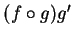. Hence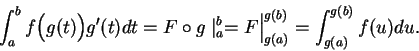Hence we can find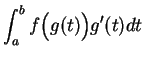by the following ritual:

Let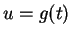. When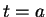then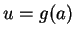and when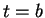then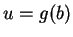. Also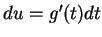. Hence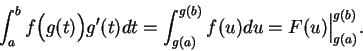17.51   Example. To find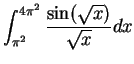.

Let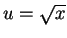. When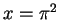, then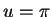, and when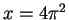, then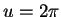. Also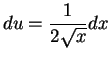, so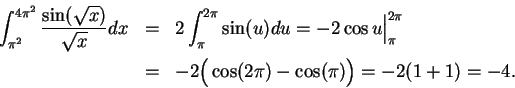We saw in (17.36) that if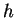is an inverse function for, then an antiderivative for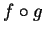is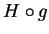, where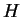is an antiderivative for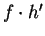. Thus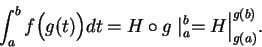The ritual for finding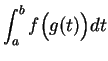in this case is:

Let. Then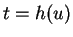and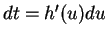. Whenthen, and whenthen. Thus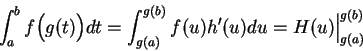whereis an antiderivative for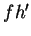.

17.52   Example. To find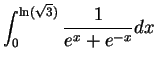.

Let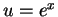. When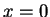then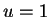, and when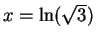then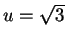. Also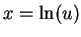, so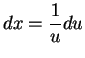.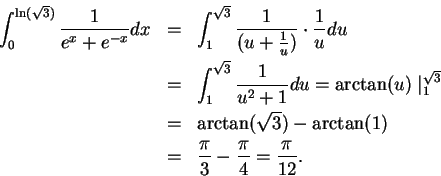17.53   Exercise. A Find the following integrals:

a)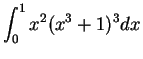.

b)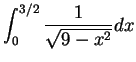.

c)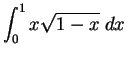.

17.54   Exercise. A Find the area of the shaded region, bounded by the ellipse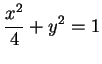and the lines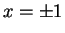.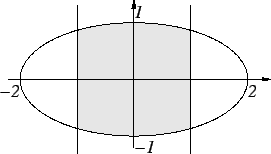17.55   Example. In practice I would find many of the antiderivatives and integrals discussed in this chapter by computer. For example, using Maple, I would find

> int(sqrt(a^2+x^2),x);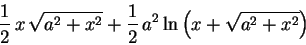> int(sin(sqrt(x)),x=0..Pi^2);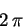> int(sqrt(4 - x^2),x=-1..1);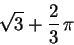> int( (sec(x))^3,x);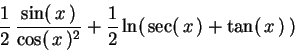> int(exp(a*x)*cos(b*x),x);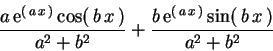Next: 17.7 Rational Functions Up: 17. Antidifferentiation Techniques Previous: 17.5 Trigonometric Substitution   Index
Ray Mayer 2007-09-07